RRB JE EEE (CBT I) Mock Test- 5

# RRB JE EEE (CBT I) Mock Test- 5

Test Description

## 100 Questions MCQ Test RRB JE Mock Test Series Electrical & Electronics Engineering | RRB JE EEE (CBT I) Mock Test- 5

RRB JE EEE (CBT I) Mock Test- 5 for Railways 2022 is part of RRB JE Mock Test Series Electrical & Electronics Engineering preparation. The RRB JE EEE (CBT I) Mock Test- 5 questions and answers have been prepared according to the Railways exam syllabus.The RRB JE EEE (CBT I) Mock Test- 5 MCQs are made for Railways 2022 Exam. Find important definitions, questions, notes, meanings, examples, exercises, MCQs and online tests for RRB JE EEE (CBT I) Mock Test- 5 below.
Solutions of RRB JE EEE (CBT I) Mock Test- 5 questions in English are available as part of our RRB JE Mock Test Series Electrical & Electronics Engineering for Railways & RRB JE EEE (CBT I) Mock Test- 5 solutions in Hindi for RRB JE Mock Test Series Electrical & Electronics Engineering course. Download more important topics, notes, lectures and mock test series for Railways Exam by signing up for free. Attempt RRB JE EEE (CBT I) Mock Test- 5 | 100 questions in 90 minutes | Mock test for Railways preparation | Free important questions MCQ to study RRB JE Mock Test Series Electrical & Electronics Engineering for Railways Exam | Download free PDF with solutions
 1 Crore+ students have signed up on EduRev. Have you?
RRB JE EEE (CBT I) Mock Test- 5 - Question 1

### Solve the following expression: 452 - 35 * 68 - 58 + 60% of 730.

Detailed Solution for RRB JE EEE (CBT I) Mock Test- 5 - Question 1

= 452 - 35 * 68 - 58 + 60% of 730

= 2025 - 2380 - 58 + 438

= 2463 - 2438

= 25

= 52

RRB JE EEE (CBT I) Mock Test- 5 - Question 2

### The angles of elevation of the top of a tower 90 m high, from two points on the level ground on its opposite sides are 45° and 60°. What is the distance between the two points (rounded off to the nearest integer)? (√3 = 1.732)

Detailed Solution for RRB JE EEE (CBT I) Mock Test- 5 - Question 2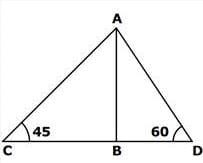Let AB be the tower and C and D the two points.

Now AB/BC = tan 45° = 1 AB=BC=90

Also AB/BD = tan 60° = V3 BD = 90/% 3 = 30%3

Hence CD = CB+BD = 90+30\3 = 90 + 51.96 = 141.96 ≈ 142 m

RRB JE EEE (CBT I) Mock Test- 5 - Question 3

### Two quadratic equations: 16x2 - Ax + 25 and Bx2 + Ax + 4 = 0 have real and equal roots, then what is the value of √B?

Detailed Solution for RRB JE EEE (CBT I) Mock Test- 5 - Question 3

16x2 - Ax + 25 has real and equal roots

(-A)2 = 4 * 16 * 25

A = 40

Bx2 + Ax + 4 = 0 has real and equal roots

(A)2 = 4 * B * 4

(40)2 = 4 * B * 4

B = 100

√B = √100

√B = 10

RRB JE EEE (CBT I) Mock Test- 5 - Question 4

Roshan deposited Rs 17500 in the bank which offers compound interest at 8% per annum. What is the interest obtained on the sum after 2 years?

Detailed Solution for RRB JE EEE (CBT I) Mock Test- 5 - Question 4 Amount after two years = 17500 x (1+8/100)2

= 17500 x 1.08 x 1.08 = 20412

Interest = 20412 - 17500 = Rs 2912

RRB JE EEE (CBT I) Mock Test- 5 - Question 5

Length of a rectangle is increased by 10%, and breadth is decreased by 10%. Find the percent effect in the area.

Detailed Solution for RRB JE EEE (CBT I) Mock Test- 5 - Question 5 Let length = l

And breadth = b

Area = lb

After increment, length = l x (100 + 10)/100 = l x 110/100 = l x 11/10

After decrement, breadth = b x (100 - 10)/100 = b x 90/100 = b x 9/10

Area = l x 11/10 x b x 9/10

= 99lb/100 lb

% decrease = (lb - 99lb/100)/lb x 100

= (100lb - 99lb)/100 x 1/lb x 100

= lb/100 x 1/lb x 100

= 1%

RRB JE EEE (CBT I) Mock Test- 5 - Question 6

One of the angles of a triangle is half the larger angle of a parallelogram. The respective ratio between the adjacent angles of the parallelogram is 14 : 31 . The smallest angle of the triangle is half the smaller angle of the parallelogram. What is the value of the largest angle of the triangle?

Detailed Solution for RRB JE EEE (CBT I) Mock Test- 5 - Question 6

We know that the sum of the adjacent angles of a parallelogram = 180o

Let the adjacent angles of the parallelogram be 14x and 31x

Therefore, 14x + 31x = 180o

45x = 180o

X = 4

RRB JE EEE (CBT I) Mock Test- 5 - Question 7

Aman donated 10% of his Savings to Charity and gave 15% of his savings to his sister. Of the remaining savings his gave 60% to his son and gave 80% of the still remaining saving to his daughter. If the sum of the money given to sister and the money left with Aman finally is Rs 84,000, what was his savings?

Detailed Solution for RRB JE EEE (CBT I) Mock Test- 5 - Question 7

Let his savings be Rs 100x

Money donated to charity = Rs 10x and Money given to sister = Rs 15x

Money left with Aman = 100x - 10x - 15x = Rs 75x

Money left with him after giving to son = (40/100)*75x = Rs 30x

Money left with him after giving to daughter = (20/100)*30x = Rs 6x

Given, 15x + 6x = 84000

⇒ 21x = 84000

⇒ x = 4000

His Savings = Rs 4,00,000

RRB JE EEE (CBT I) Mock Test- 5 - Question 8

The ratio of 4th and 2nd term of an A.P of positive terms is 5:3 and the product of the 1st and the 3rd terms is 72. what is the product of the 5th and 6th terms of the AP?

Detailed Solution for RRB JE EEE (CBT I) Mock Test- 5 - Question 8 Let the first term be 'a' and the common difference be 'd'.

Given, (a + 3d)/(a + d) = 5/3

⇒ 3a + 9d = 5a + 5d

⇒ 2a = 4d

⇒ a = 2d

Also, a(a + 2d) = 72

⇒ 2d(2d + 2d) = 72

⇒ 8d2 = 72

⇒ d2 = 9

⇒ d = 3

∴ a = 2*3 = 6

d ≠ −3 as the 1st term will become a = 2(−3) = − 6 but all terms are positive.

5th term = a + 4d = 6 + 4*3 = 18

6th term = 18 + 3 = 21

Required product = 18*21 = 378

RRB JE EEE (CBT I) Mock Test- 5 - Question 9

Three pipes A, B, and C can fill a cistern in 10 hrs. After working together for 4 hours, C is closed, and A and B fill the cistern in 9 hrs. Then find the time in which the cistern can be filled by pipe C?

Detailed Solution for RRB JE EEE (CBT I) Mock Test- 5 - Question 9 A, B, and C can fill in 1 hour = 1/10 of the cistern

A, B, and C can fill in 4 hour= 4/10 =2/5 of the cistern

Remaining part = 1 - 2/5 =3/5 of cistern

3/5 of the cistern is filled by A+B in 9 hours

Therefore, A and B can fill the cistern in 9*5/3 = 15 hrs.

Hence in one hour C filled the cistern = 1/10 - 1/15 = 1/30 of the cistern

Hence C can fill the cistern in 30 hrs.

RRB JE EEE (CBT I) Mock Test- 5 - Question 10

If the average of twelve consecutive even numbers is 113, find the sum of the 3rd and 8th numbers among them?

Detailed Solution for RRB JE EEE (CBT I) Mock Test- 5 - Question 10 Let the 1st number = x

According to the question,

x + (x+2) + (x+4) + ...... + (x+22) = 113*12

12x + 132 = 1356

12x = 1224

x = 102

Therefore 3rd number = 102 + 4 = 106

8th number = 102 + 14 = 116

Hence the required answer = 106 + 116 = 222

RRB JE EEE (CBT I) Mock Test- 5 - Question 11

What approximate value should come in the place of the question mark in the following question?

72)2 ÷ ∛46656=?

Detailed Solution for RRB JE EEE (CBT I) Mock Test- 5 - Question 11 ? = (72)2 ÷ ∛46656

? = (72)2 ÷ ∛(36x36x36) = (72)2 ÷ 36

? = (72 X 72)/36 = 144

RRB JE EEE (CBT I) Mock Test- 5 - Question 12

Ranjan purchases an article at Rs.660 and marks up the price by 25%, and after allowing the discount of Rs.99, he gains __% profit on the sale.

Given below are the steps involved. Arrange them in sequential order.

A) Per cent profit = (66/660) * 100 = 10%

B) Selling price of the item after discount of Rs.99 = 825 - 99 = Rs.726

C) Profit amount earned on the item = 726 - 660 = Rs.66

D) Cost price of the article is Rs.660 and marked price = 125% of 660 = Rs.825

Detailed Solution for RRB JE EEE (CBT I) Mock Test- 5 - Question 12 The correct arrangement is

D) Cost price of the article is Rs.660 and marked price = 125% of 660 = Rs.825B) Selling price of the item after discount of Rs.99 = 825 - 99 = Rs.726

C) Profit amount earned on the item = 726 - 660 = Rs.66

A) Per cent profit = (66/660) * 100 = 10%

RRB JE EEE (CBT I) Mock Test- 5 - Question 13

Study the following table carefully and answer the related question.

Following table represents the three types of products A, B and C manufactured in a company during Five months.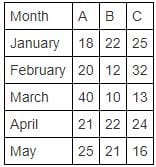In which of the following month there is a maximum percentage increase/decrease in the manufacturing of all three types of products together?

Detailed Solution for RRB JE EEE (CBT I) Mock Test- 5 - Question 13

Total products manufactured:

In January = 18 + 22 + 25 = 65

In February = 20 + 12 + 32 = 64

In March = 40 + 10 + 13 = 63

In April = 21 + 22 + 24 = 67

In May = 25 + 21 + 16 = 62

Percentage increase/decrease:

In February = ((65 - 64)/65) x 100 = 1.54%

In March = ((64 - 63)/64) x 100 = 1.56%

In April = ((67 - 63)/63) x 100 = 6.35%

In May = ((67 - 62)/67) x 100 = 7.46%

RRB JE EEE (CBT I) Mock Test- 5 - Question 14

Two trains 140 m and 160 m long run at the speed of 60 kmph and 40 kmph respectively in opposite directions on parallel tracks. The time (in seconds) which they take to cross each other is :

Detailed Solution for RRB JE EEE (CBT I) Mock Test- 5 - Question 14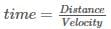Distance = 140 + 160 = 300m = 0.3 km

Relative velocity = 60 + 40 = 100 kmph (opp. direction)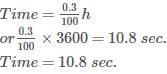RRB JE EEE (CBT I) Mock Test- 5 - Question 15

a2+b2=5 and a3+b3=9. If ab = 2, what is the value of a4+b4?

Detailed Solution for RRB JE EEE (CBT I) Mock Test- 5 - Question 15

Let (a+b) = c

c2 = (a+b)2 = a2+b2 + 2ab = 5 + 4 = 9

⇒ a + b = 3

Now, (a+b)(a3+b3) = a4+b4+ab(a2+b2)

⇒ 3 x 9 = a4+b4 + 2 x 5

⇒ a4+b4 = 17

RRB JE EEE (CBT I) Mock Test- 5 - Question 16

The average weight of 24 students in a class is 40 kg. if the weight of the teacher is included, the average is increased by 500 gms. Find The weight of the teacher?

Detailed Solution for RRB JE EEE (CBT I) Mock Test- 5 - Question 16 25*40.5 - 24*40 = 52.5

Hence, the answer is option (2).

RRB JE EEE (CBT I) Mock Test- 5 - Question 17

A shopkeeper makes a profit of 20% by selling a T.V. What would be the new profit percent if the shopkeeper paid 15% less for the T.V and the customer paid 10% more for the T.V?

Detailed Solution for RRB JE EEE (CBT I) Mock Test- 5 - Question 17 Let the price at which T.V was purchased by shopkeeper = Rs 100a

Hence the price at which T.V was sold by shopkeeper = Rs 120a

If shopkeeper paid 15% less than the previous amount hence new cost price of the T.V = Rs (100a X 0.85) = Rs 85a

New selling price of the T.V = Rs (120a X 1.1) = Rs 132a

Profit % = (132a-85a)/85a X 100 = 55.3%

RRB JE EEE (CBT I) Mock Test- 5 - Question 18

Solve the following expression: (13/8) of (15/32) of 45% of 3072.

Detailed Solution for RRB JE EEE (CBT I) Mock Test- 5 - Question 18

= (13/8) of (15/32) of 45% of 3072

= (13/8) * (15/32) * (45/100) * 3072

= (13/8) * (3/32) * (9/4) * 307

= 13 * 3 * 9 * 3

= 1053

RRB JE EEE (CBT I) Mock Test- 5 - Question 19

Following Pie Chart shows the percentage distribution of people of different ages in a Building. The table shows the ratio of Male and Female people in each age group. There is a total of 800 people in the building.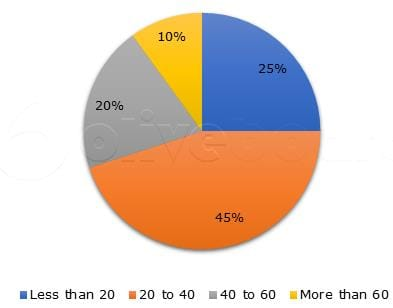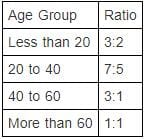What is the difference between the total number of Males of age 20 to 60 and the total number of Females of age 20 to 60?

Detailed Solution for RRB JE EEE (CBT I) Mock Test- 5 - Question 19 Calculating the number of Male and Females of each age group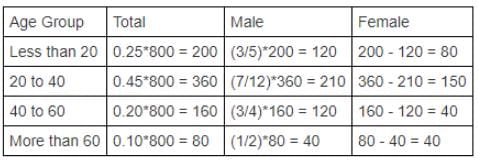total number of Males of age 20 to 60 = 210 + 120 = 330

total number of Females of age 20 to 60 = 150 + 40 = 190

Required difference = 330 - 190 = 140

RRB JE EEE (CBT I) Mock Test- 5 - Question 20

The population of a village is 6000. The next year, the number of male increases by 10% and the number of female increases by 20% and the population becomes 6840. What is the initial population of Male?

Detailed Solution for RRB JE EEE (CBT I) Mock Test- 5 - Question 20 Let the initial population of Males and Females be 100x and 100y respectively.

Given, 100x + 100y = 6000

⇒ x + y = 60..(i)

And, 110x + 120y = 6840

⇒ 11x + 12y = 684..(ii)

12*(i) - (ii), gives

x = 36

Initial population of Males = 3600

RRB JE EEE (CBT I) Mock Test- 5 - Question 21

The average age of a Sheela and her sister is 16 years. The respective ratio of their ages is 7:1 respectively. Find Sheela's age?

Detailed Solution for RRB JE EEE (CBT I) Mock Test- 5 - Question 21 Sum of their ages = 2 X 16 = 32

Let 7x and x be their respective ages, then, 8x = 32 and x = 4

So, Sheela's age = 7x = 7 X 4 = 28 years . Hence, option 1.

RRB JE EEE (CBT I) Mock Test- 5 - Question 22

20% of x% of 60 is equal to y% of 60% of 40, what is the value of the ratio x:y?

Detailed Solution for RRB JE EEE (CBT I) Mock Test- 5 - Question 22 20% of x% of 60 = y% of 60% of 40

x = 2y

x:y = 2:1

RRB JE EEE (CBT I) Mock Test- 5 - Question 23

What is the remainder when x20 + x19 + x18 + x17 + x16 + x15 + x14 + x13 + x12 + x11 is divided by x9 + x8 + x7 + x6 + x5 + x4 + x3 + x2 + x + 1

Detailed Solution for RRB JE EEE (CBT I) Mock Test- 5 - Question 23 Numerator = x11 (x9 + x8 + x7 + x6 + x5 + x4 + x3 + x2 + x + 1) = x11 times the denominator.

The denominator divides the numerator, so the answer is 0.

RRB JE EEE (CBT I) Mock Test- 5 - Question 24

A person invested equal amounts in two scheme A and B at the same rate of interest. Scheme A offers simple interest while scheme B offers compound interest. After two years he got Rs. 1920 from the Scheme A as interest and Rs. 2112 from scheme B. If the rate of interest is increased by 4%, what will be the total interest after two years both schemes ?

Detailed Solution for RRB JE EEE (CBT I) Mock Test- 5 - Question 24 C.I - S.I= 192

S.I for 1 year = 960

Interest on RS 960 for 1 year is 192

so rate is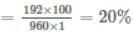so the principal is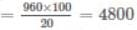when interest is increased is 4% then new rate of interest is 24%

So simple interest after two years will be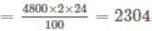and compound interest rate after two years will be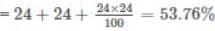Compound interest after two years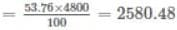So total interest is = 2304+2580.48 = Rs. 4884.48

RRB JE EEE (CBT I) Mock Test- 5 - Question 25

How many litres of a solution containing milk and water in the ratio 2:3 should be added to 30 litres of 70 % milk solution in order to get 60% milk solution?

Detailed Solution for RRB JE EEE (CBT I) Mock Test- 5 - Question 25 Let the number of liters of solution added be: x

The initial ratio of milk (m) to water (w) is:

⇒ m/w = ⅔

⇒ m = 2/5 of the solution

⇒ m = 40% of the solution

Acc. to the question, after adding x liters of 40% milk solution to 30 liters of 70% milk solution, we get 60% of (x+30) liters milk solution.

Hence, the equation becomes:

⇒ 40x + 30 * 70 = (x+30) * 60

⇒ 40x + 2100= 60x + 1800

⇒ 20x = 300

⇒ x = 15

RRB JE EEE (CBT I) Mock Test- 5 - Question 26

If 12% of a is equal to 15% of b. Find the respective ratio of a and b.

Detailed Solution for RRB JE EEE (CBT I) Mock Test- 5 - Question 26 12% of a = 15% of b

⇒ a/b = 15/12

⇒ a/b = 5/4

⇒ a:b = 5:4

RRB JE EEE (CBT I) Mock Test- 5 - Question 27

Downstream speed of boat A is 20% more than the downstream speed of boat B whose upstream speed is 6 km/hr less than upstream speed of boat A. What is the speed of boat A in still water? The stream for both boat is moving with 10 km/hr.

Detailed Solution for RRB JE EEE (CBT I) Mock Test- 5 - Question 27 Stream speed = 10 km/hr

Downstream speed of boat A = (6/5) x downstream speed of boat B

Upstream speed of boat A - upstream speed of boat B = downstream speed of boat A - downstream speed of boat B = 6

(6/5) x downstream speed of boat B - downstream speed of boat B = 6

Downstream speed of boat B = 30 km/hr

Downstream speed of boat A = 30 + 6 = 36 km/hr

Speed of boat A in still water = 36 - 10 = 26 km/hr

RRB JE EEE (CBT I) Mock Test- 5 - Question 28

If monthly income of a person is increased by 20% and his expenditures are increased by 40%, then his savings are decreased by what percent? (ratio of income and expenditure is 4: 3 respectively.)

Detailed Solution for RRB JE EEE (CBT I) Mock Test- 5 - Question 28 Let income and expenditure are 'a' and 'b' respectively.

Savings = a - b = a - 3a/4 = a/4

When income and expenditure are increased by 20%. Then,

New income = 120% of a = 1.2a

New expenditure = 140% of b = 1.4 x 3a/4 = 2.1a/2

New savings = 1.2a - 2.1a/2 = 3a/20

Decrement in savings = (a/4) - (3a/20) = a/10

Percentage = a/10)/(a/4 x 100 = 40%

RRB JE EEE (CBT I) Mock Test- 5 - Question 29

A and B can finish a work in 2 days, B and C in 3 days and A and C in 4 days. Find the ratio of time taken by A alone and C alone to finish the work?

Detailed Solution for RRB JE EEE (CBT I) Mock Test- 5 - Question 29 Suppose A, B and C take A, B and C days respectively to finish the work on their own.

1/A + 1/B = 1/2 (1)

1/B + 1/C = 1/3 (2)

1/A + 1/C = 1/4 (3)

(1)+(2)+(3) ⇒ 2(1/A+1/B+1/C) = 13/12

⇒ 1/A + 1/B + 1/C = 13/24 (4)

(4) - (2) ⇒ 1/A = 5/24

Thus A takes 24/5 = 4.8 days.

(4) - (1) ⇒ 1/C = 1/24

Hence C takes 24 days.

Required ratio = 4.8: 24 = 1:5

RRB JE EEE (CBT I) Mock Test- 5 - Question 30

Which of the following is irrational?

Detailed Solution for RRB JE EEE (CBT I) Mock Test- 5 - Question 30 Product of (3 - √27) and (√9 + 3√3)

= (3 - 3√3)(3 + 3√3)

= - 18 (which is not irrational)

Option (2):

= Sum of (5 - 4√2) and 4(6 + √2)

= (5 - 4√2) + 4(6 + √2)

= 5 - 4√2 + 24 + 4√2

= 29 (which is not irrational)

Option (3):

= Sum of √4761 and √5175

= 69 + 15√23 (which is irrational)

Option (4):

= Product of √16875 and √27

= 75√3 x 3√3

= 675 (which is not irrational)

RRB JE EEE (CBT I) Mock Test- 5 - Question 31

Pointing to a boy in a photograph Akhil said "He is the son of the only son of my mother". How is Akhil related to that boy?

Detailed Solution for RRB JE EEE (CBT I) Mock Test- 5 - Question 31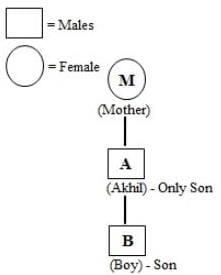RRB JE EEE (CBT I) Mock Test- 5 - Question 32

If’ A’ is coded as 1, ‘B’ as 3, ‘C’ as 5 and so on, which of the following is the numerical value of the word ‘FAZED’?

Detailed Solution for RRB JE EEE (CBT I) Mock Test- 5 - Question 32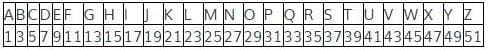So from the above table, numerical value of FAZED = 11 + 1 + 51 + 9 + 7 = 79.

RRB JE EEE (CBT I) Mock Test- 5 - Question 33

DIRECTIONS: In the given question find the answer figure in which question is embedded.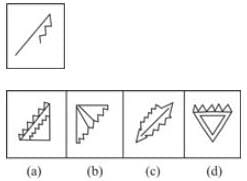Detailed Solution for RRB JE EEE (CBT I) Mock Test- 5 - Question 33 figure (a)
RRB JE EEE (CBT I) Mock Test- 5 - Question 34

DIRECTIONS: Read the following information carefully to answers the questions that follow.

Two brothers Manin and Nitin walk in opposite directions for 3km. Manin is walking towards East.After 3km each, both turn right and again walk 3 km each. Both turn to face each other.

In which direction is Nitin looking?

Detailed Solution for RRB JE EEE (CBT I) Mock Test- 5 - Question 34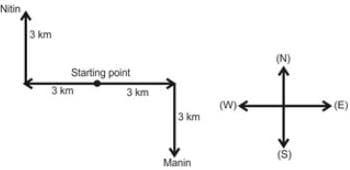From the above diagram, it is clear that, Nitin is looking in South-East direction.

RRB JE EEE (CBT I) Mock Test- 5 - Question 35

In the given sequence how many such symbols and numbers are there which are either immediately preceded or immediately followed by the letters which is from the first half of the English alphabet?

2 L K @ 8 \$ P B 1 V # 6 % G W 9 J C D 4 © 7 F R 4 A

Detailed Solution for RRB JE EEE (CBT I) Mock Test- 5 - Question 35 Such combinations are

2L, K@, B1, %G, 9J, D4, 7F, 4A,

RRB JE EEE (CBT I) Mock Test- 5 - Question 36

Select the odd letter/word/number from the given alternatives.

Detailed Solution for RRB JE EEE (CBT I) Mock Test- 5 - Question 36 All others are the drinks.
RRB JE EEE (CBT I) Mock Test- 5 - Question 37

DIRECTIONS: Each of the following questions consists of five figures marked A, B, C, D and E if called the Problem figures followed by five other figures marked 1, 2, 3 and 4 called the Answer Figures. Select a figure from amongst the Answer Figures which will continue the same series as established by the five Problem Figures.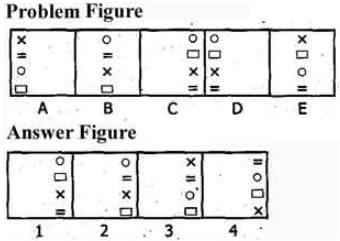Detailed Solution for RRB JE EEE (CBT I) Mock Test- 5 - Question 37 All the symbols move horizontally. In the first step, the first and third symbols interchange positions. In the second step, the second and fourth symbols interchange positions. In the third step, the symbols remain as such. The Whole process is then repeated.
RRB JE EEE (CBT I) Mock Test- 5 - Question 38

DIRECTIONS: Each of the following questions consists of five figures marked A, B, C, D and E called the Problem Figures followed by five other figures marked 1, 2, 3 and 4 called the Answer figures. Select a figure from amongst the Answer Figures which will continue the same series as established by the five Problem Figures.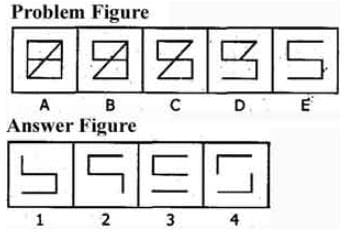Detailed Solution for RRB JE EEE (CBT I) Mock Test- 5 - Question 38 One line segment disappears from the figure In each step, one from the lower portion and one from the upper portion alternately.
RRB JE EEE (CBT I) Mock Test- 5 - Question 39

Choose the odd one out from the given alternatives.

Detailed Solution for RRB JE EEE (CBT I) Mock Test- 5 - Question 39 First number ÷ Second number = Remainder 2

26/3 = Remainder 2

39/4 = Remainder 3

42/5= Remainder 2

44/6 = Remainder 2

RRB JE EEE (CBT I) Mock Test- 5 - Question 40

In a certain code language, ‘nick muk pic’ means ‘brave and concern’, ‘ill dic so’ means ‘every body else’ and ‘tur muk so’ means ‘body and soul’. Which of the following may mean ‘and every soul’?

Detailed Solution for RRB JE EEE (CBT I) Mock Test- 5 - Question 40

From (1) & (3)

muk = and

From (2) & (3)

so = body

From (3)

soul = tur

From (2)

every / else = ill/dic

From (1)

concern/brave = nick / pic

So, ‘and every soul’ may be ‘muk tur dic’,.

RRB JE EEE (CBT I) Mock Test- 5 - Question 41

Rajitha walked 80 m before taking a left turn. She then walked 60 m and took a left turn to walk another 50 m. Finally she took a right turn and walked 30 m to reach the bank, now if she faces East, in which direction did she start walking?

Detailed Solution for RRB JE EEE (CBT I) Mock Test- 5 - Question 41 The path traced by Rajitha is as follows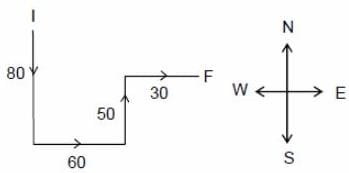As Rajitha was facing East at the end which implies that initially she started walking in the South direction.

RRB JE EEE (CBT I) Mock Test- 5 - Question 42

DIRECTIONS: In each of the following questions, choose the set of numbers out of the four options that is similar to the given set.

given set: (21, 51, 15)

Detailed Solution for RRB JE EEE (CBT I) Mock Test- 5 - Question 42 Middle numbers = first number + 2 × second number.
RRB JE EEE (CBT I) Mock Test- 5 - Question 43

DIRECTIONS: In each of the following questions, choose the set of numbers out of the four options that is similar to the given set.

Given set : (63, 8, 3)

Detailed Solution for RRB JE EEE (CBT I) Mock Test- 5 - Question 43 Add 1 to first number and take the square root to find the second number, and similarly with the 2nd number we will find 3rd number
RRB JE EEE (CBT I) Mock Test- 5 - Question 44

DIRECTIONS: In the following question, continuous pattern series is given. Some of the letters of the series are missing. These missing letters are given in that order as one of the four alternatives below the series. Find out the correct alternatives.

Detailed Solution for RRB JE EEE (CBT I) Mock Test- 5 - Question 44 In the above series, the letters are equidistant from the beginning and the end.

Hence, the missing letter are cbbaa.

RRB JE EEE (CBT I) Mock Test- 5 - Question 45

DIRECTIONS: In the question below, there are few statements followed by few conclusions. You have to take the given statements to be true even if they seem to be at variance with commonly known facts and then decide which of the given conclusion logically follow(s) from the given statements.

Statements: All fruits are lions.

All lions are foxes.

Some foxes are elephants.

Conclusions:

I. All fruits are foxes.

II. Some fruits are elephants.

Detailed Solution for RRB JE EEE (CBT I) Mock Test- 5 - Question 45 Only Conclusion I follows.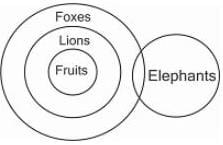RRB JE EEE (CBT I) Mock Test- 5 - Question 46

DIRECTIONS: Study the venn diagram to answer the given question.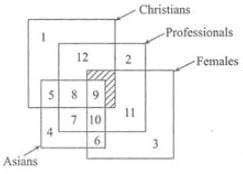Asian non-Christian females who are professionals are represented by-

Detailed Solution for RRB JE EEE (CBT I) Mock Test- 5 - Question 46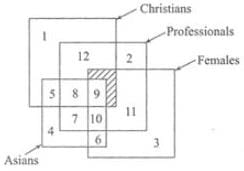RRB JE EEE (CBT I) Mock Test- 5 - Question 47

Pointing to a man in the photograph a woman says: “He is the father of my daughter-in-law’s brother-in-law.” How is the man related to the woman?

Detailed Solution for RRB JE EEE (CBT I) Mock Test- 5 - Question 47 Daughter-in-law’s brother-in-law = Son.

Father of son = Husband.

RRB JE EEE (CBT I) Mock Test- 5 - Question 48

If B is coded as 8, F is coded as 6, Q is coded as 4, D is coded as 7, T is coded as 2, M is coded as 3, and K is coded as 5, then what is the coded form of QKTBFM?

Detailed Solution for RRB JE EEE (CBT I) Mock Test- 5 - Question 48 Q K T B F M = 4 5 2 8 6 3
RRB JE EEE (CBT I) Mock Test- 5 - Question 49

Which of the words in the following options can be formed using the given word ?

DETERMINATION

Detailed Solution for RRB JE EEE (CBT I) Mock Test- 5 - Question 49 There is no ‘C’ in the given word.So, DECLARATION cannot be formed.

There is no ‘L’ in the given the word. So, NATIONAL cannot be formed.

There is no ‘V’ in the given word.So, DEVIATION cannot be formed.

RRB JE EEE (CBT I) Mock Test- 5 - Question 50

DIRECTIONS: Arrange the following in a logical order.

1. Puberty

3. Childhood

4. Infancy

5. Senescence

Detailed Solution for RRB JE EEE (CBT I) Mock Test- 5 - Question 50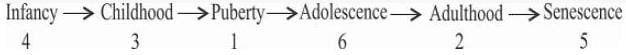RRB JE EEE (CBT I) Mock Test- 5 - Question 51

Cattle, cows and oxen can be represented by

Detailed Solution for RRB JE EEE (CBT I) Mock Test- 5 - Question 51 Cow and oxen both are cattle.
RRB JE EEE (CBT I) Mock Test- 5 - Question 52

The day on 18.09.1977 was Sunday. A couple was married on this date. In the next 15 years, there how many marriage anniversaries would fall on Sunday?

Detailed Solution for RRB JE EEE (CBT I) Mock Test- 5 - Question 52 1977 is an ordinary year. We know that the calendar of an ordinary year repeats after 6 yrs or 11 yrs.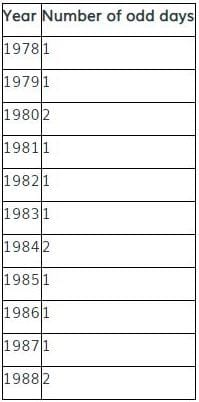Therefore,

Number of odd days from 18.09.1977 to 18.09.1983=7, i.e., 0 odd day

It means that in 1983, 18th September would fall on Sunday.

and number of odd days from 18.09.1977 to 18.09.1988 = 14, i.e., 0 odd day.

Now, it is clear that 2 marriage anniversaries would fall on Sunday in the next 15 yr.

RRB JE EEE (CBT I) Mock Test- 5 - Question 53

In a queue, Lily is eighteenth from the front while Mini is sixteenth from the back .If Nisha is twenty- fifth from the front and is exactly in the middle of Lily and Mini, then how many persons are there in the queue?

Detailed Solution for RRB JE EEE (CBT I) Mock Test- 5 - Question 53 Lily is 18th from front and Nisha is 25th .

Number of persons between Lily and Nisha = 6.

Since Nisha is exactly in middle of Lily and Mini, so number of persons between Nisha and Mini = 6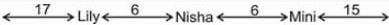∴ Number of persons in the queue = (17 + 1 + 6 + 1 + 6 + 1 + 15) = 47.

RRB JE EEE (CBT I) Mock Test- 5 - Question 54

DIRECTIONS: In the question below, which character when placed at the sign of interrogation shall complete the matrix?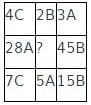Detailed Solution for RRB JE EEE (CBT I) Mock Test- 5 - Question 54

In each row, out of the letters A, B and C, each of these must appear once. In each column, the product of the first and third numbers is equal to the second number. So, the missing number will be (2 × 5) i.e.,10 and the letter will be C.

Thus, the answer is 10C.

RRB JE EEE (CBT I) Mock Test- 5 - Question 55

DIRECTIONS : Given below are two Matrices of Twenty-five Cells, each containing two classes of alphabets. The columns and rows of Matrix I are numbered form 0 to 4 and that of Matrix II from 5 to 9. A letter from these Matrices can be represented first by its row number and next by its column number. In each of the following questions, identify one set of number pairs out of (1), (2), (3) and (4) which represents the given word.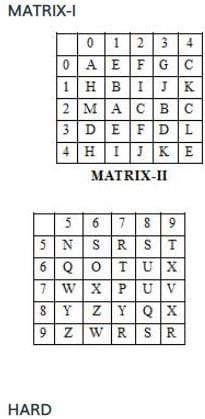Detailed Solution for RRB JE EEE (CBT I) Mock Test- 5 - Question 55

HARD

H– 10, 40

A– 00, 21

R– 57, 97, 99

D– 30, 33

Therefore, the correct answer is option (3) i.e. 40, 21, 57, 33

RRB JE EEE (CBT I) Mock Test- 5 - Question 56

In which of the following Indian state are you likely to see Salher range?

Detailed Solution for RRB JE EEE (CBT I) Mock Test- 5 - Question 56

Salher range is a part of western ghat with its extension in Maharshtra.

RRB JE EEE (CBT I) Mock Test- 5 - Question 57

Finance Commisson is included in________of Indian Constitution.

Detailed Solution for RRB JE EEE (CBT I) Mock Test- 5 - Question 57 The Finance Commission came into existence in 1951. It was established under Article 280 of the Indian Constitution by the President of India.
RRB JE EEE (CBT I) Mock Test- 5 - Question 58

In a code language, A is written as B, B is written as C, C is written as D and so on, then how will STUDY be written in that code language ?

Detailed Solution for RRB JE EEE (CBT I) Mock Test- 5 - Question 58 The letters are coded by moving them 1 step forward.
RRB JE EEE (CBT I) Mock Test- 5 - Question 59

Nallamalai, Veliconda are associated with which of the following?

Detailed Solution for RRB JE EEE (CBT I) Mock Test- 5 - Question 59 The Nallamalas (also called the Nallamalla Range) are the section of the Eastern Ghats which stretch primarily over Kurnool, Nellore, Guntur, Prakasam, Kadapa and Chittoor districts of the state of Andhra Pradesh and Mahabubnagar, Nalgonda districts of the state of Telangana, in India.
RRB JE EEE (CBT I) Mock Test- 5 - Question 60

What is the rank of India in terms of genetically modified crops worldwide?

Detailed Solution for RRB JE EEE (CBT I) Mock Test- 5 - Question 60 Indian ranked fifth in terms of area in genetically modified crops worldwide.
RRB JE EEE (CBT I) Mock Test- 5 - Question 61

Who was the British Governor General during Third Anglo-Mysore War?

Detailed Solution for RRB JE EEE (CBT I) Mock Test- 5 - Question 61 Lord Cornwallis was the British Governor General during Third Anglo-Mysore War.
RRB JE EEE (CBT I) Mock Test- 5 - Question 62

When was Civil Disobedience withdrawn?

Detailed Solution for RRB JE EEE (CBT I) Mock Test- 5 - Question 62 The Civil Disobedience movement was withdrawn in May 1934.
RRB JE EEE (CBT I) Mock Test- 5 - Question 63

Under which Article of the constitution of India, can the fundamental rights of member of the Armed Forces be specially restricted ?

Detailed Solution for RRB JE EEE (CBT I) Mock Test- 5 - Question 63 Article 33 & 34 empower the Parliament to restrict, modify or abrogate the fundamental rights to the members of armed forces, para-military forces, police forces, members of intelligence agencies or similar services.
RRB JE EEE (CBT I) Mock Test- 5 - Question 64

If the posts of the President and Vice-president fall vacant, who will act as the President of India?

Detailed Solution for RRB JE EEE (CBT I) Mock Test- 5 - Question 64 If the post of president and vice president falls vacant, then the chief justice of India will act as the president of India as prescribed in the constitution of India. If the chief justice is also not present, then the senior-most judge of the supreme court will act as president.

This Precedence has happened only once in the Indian Political timeline when Md. Hidayatullah (then CJI) became President after the death of Zakir Hussain and the subsequent resignation of VV Giri in 1969.

RRB JE EEE (CBT I) Mock Test- 5 - Question 65

Lala Lajpat Rai was associated with the following newspapers-

1. Kohinoor

2. The Punjabi

3. Vande Mataram

4. The Pupil

Detailed Solution for RRB JE EEE (CBT I) Mock Test- 5 - Question 65 Both the Pupil and Punjabi newspaper were the started by Lala Lajpat Rai, whereas, Vande Matram is started by Bipin Chandra Pal and Kohinoor by Munshi Harsukh Rai.
RRB JE EEE (CBT I) Mock Test- 5 - Question 66

Who among the following artist has been conferred with Tansen Samman for 2018?

Detailed Solution for RRB JE EEE (CBT I) Mock Test- 5 - Question 66 Noted sitar player Manju Mehta has been conferred with the 'Tansen Samman' for 2018 by the Madhya Pradesh government for her contribution in the field of music.
RRB JE EEE (CBT I) Mock Test- 5 - Question 67

Budapest city is located on the banks of which river?

Detailed Solution for RRB JE EEE (CBT I) Mock Test- 5 - Question 67 Budapest is the perfect tourist destination in Europe. Budapest is the capital city of Hungary i.e., made up of Buda and Pest, with the Danube River flowing past them, along a stretch of 28 kilometers.
RRB JE EEE (CBT I) Mock Test- 5 - Question 68

The term polyarchy was used by Robert Dahl to describe a form of government in which?

Detailed Solution for RRB JE EEE (CBT I) Mock Test- 5 - Question 68 The term polyarchy was used by Robert Dahl to describe a form of government in whichPeople act both through the electoral system and the group process. Polyarchy, concept coined by the American political scientist Robert Dahl to denote the acquisition of democratic institutions within a political system that leads to the participation of a plurality of actors.
RRB JE EEE (CBT I) Mock Test- 5 - Question 69

Dispersion of seeds can be done by …?

Detailed Solution for RRB JE EEE (CBT I) Mock Test- 5 - Question 69 Wind, water, and animals are the mediums to carry the seed from one place to another.
RRB JE EEE (CBT I) Mock Test- 5 - Question 70

With reference to the religious practices in India, the “Sthanakvasi” sect belongs to:

Detailed Solution for RRB JE EEE (CBT I) Mock Test- 5 - Question 70 Sthānakavāsī is a sect of Śvētāmbara Jainism founded by a merchant named Lavaji in 1653 AD. It believes that idol worship is not essential in the path of soul purification and attainment of Nirvana.
RRB JE EEE (CBT I) Mock Test- 5 - Question 71

Which vitamin is prepared by our body in the presence of sunlight?

Detailed Solution for RRB JE EEE (CBT I) Mock Test- 5 - Question 71 Vitamin D.-Vitamin D can be made by our body itself. Our skin uses sunlight to produce vitamin D.
RRB JE EEE (CBT I) Mock Test- 5 - Question 72

Charle’s Law describes the relation between -

Detailed Solution for RRB JE EEE (CBT I) Mock Test- 5 - Question 72 Charle’s Law describes the relation between Volume and Temperature
RRB JE EEE (CBT I) Mock Test- 5 - Question 73

Where does the oxygen that keeps us alive come form?

Detailed Solution for RRB JE EEE (CBT I) Mock Test- 5 - Question 73 Since water and carbon dioxide are both compounds which contain oxygen, it is possible to obtain oxygen from either. The oxygen produced from photosynthesis is not released into the air. It is not until cellular respiration that oxygen is released. the oxygen comes from water. The water molecules are split at the “beginning” of photosynthesis for the electrons. These electrons eventually make their way to the electron transport chain, where oxygen is the final electon accept, and then released into the air. Scientists agree that there’s oxygen from ocean plants in very breath we take. Most of this oxygen comes from tiny ocean plants – called phytoplankton-that live near the water’s surface and drift with the currents. Like all plants, they photosynthesize-that is, they use sunlight and carbon dioxide to make food. A byproduct of photosynthesis is oxygen.
RRB JE EEE (CBT I) Mock Test- 5 - Question 74

Gamma rays were discovered by -

Detailed Solution for RRB JE EEE (CBT I) Mock Test- 5 - Question 74 It was discovered by Paul Villard
RRB JE EEE (CBT I) Mock Test- 5 - Question 75

Which of the following is an example of Newton’s Third Law?

Detailed Solution for RRB JE EEE (CBT I) Mock Test- 5 - Question 75 Newton’s Third Law states that “To every action, there is an equal and opposite reaction”. When a gun is fired, it thus recoils and while we swim we exert force on the water which exerts force on us, due to which we move forward.
RRB JE EEE (CBT I) Mock Test- 5 - Question 76

J J Thomson is credited with the discovery of -

Detailed Solution for RRB JE EEE (CBT I) Mock Test- 5 - Question 76 He had discovered electrons
RRB JE EEE (CBT I) Mock Test- 5 - Question 77

Smallpox is caused by:

Detailed Solution for RRB JE EEE (CBT I) Mock Test- 5 - Question 77 Smallpox is caused by two virus variants-Variola major and Variola minor.
RRB JE EEE (CBT I) Mock Test- 5 - Question 78

The shape of a rain drop is spherical due to

Detailed Solution for RRB JE EEE (CBT I) Mock Test- 5 - Question 78 High surface tension binds the raindrop from all corners from inside and that is why the raindrop is spherical in shape
RRB JE EEE (CBT I) Mock Test- 5 - Question 79

First biosphere reserve was established is 1986 at-

Detailed Solution for RRB JE EEE (CBT I) Mock Test- 5 - Question 79 First biosphere reserve was established is 1986 at Nilgiri .
RRB JE EEE (CBT I) Mock Test- 5 - Question 80

A boat will submerge when it displaces water equal to its own _______

Detailed Solution for RRB JE EEE (CBT I) Mock Test- 5 - Question 80 A boat will float when the weight of the water it displaces equals the weight of the boat and anything will float if it is shaped to displace its own weight of water before it reaches the point where it will submerge. Floating of the boat works on the principle of buoyancy force which is an upward force exerted by a liquid, gas or other fluid, that opposes the weight of an immersed object. In a column of fluid, pressure increases with depth as a result of the weight of the overlying fluid. Thus a column of fluid, or an object submerged in the fluid, experiences greater pressure at the bottom of the column than at the top. This difference in pressure results in a net force that tends to accelerate an object upwards.
RRB JE EEE (CBT I) Mock Test- 5 - Question 81

Which instrument is used to measure wind speed?

Detailed Solution for RRB JE EEE (CBT I) Mock Test- 5 - Question 81 Anemometer is a measuring instrument used in meteorology to measure wind speed. The first anemometer was invented by Leon Battista Alberti in 1450.
RRB JE EEE (CBT I) Mock Test- 5 - Question 82

Scurvy is caused by the deficiency of?

Detailed Solution for RRB JE EEE (CBT I) Mock Test- 5 - Question 82 Scurvy is caused by the deficiency of Vitamin C
RRB JE EEE (CBT I) Mock Test- 5 - Question 83

Diamond is -

Detailed Solution for RRB JE EEE (CBT I) Mock Test- 5 - Question 83 Diamond is an element.
RRB JE EEE (CBT I) Mock Test- 5 - Question 84

Friction is a

Detailed Solution for RRB JE EEE (CBT I) Mock Test- 5 - Question 84 The force responsible for changing the state of motion of objects in contact is called the force of friction. It always acts on all moving objects.
RRB JE EEE (CBT I) Mock Test- 5 - Question 85

The measurement of the gravitational pull on an object is its

Detailed Solution for RRB JE EEE (CBT I) Mock Test- 5 - Question 85 weight-the measurement of the gravitational pull on an object is its weight.
RRB JE EEE (CBT I) Mock Test- 5 - Question 86

Two richest known sources of edible protein are _______.

Detailed Solution for RRB JE EEE (CBT I) Mock Test- 5 - Question 86 The richest sources of protein are animal foods such as chicken, meat, fish, cheese and eggs. However, plant proteins are believed to be healthier because of their lower fat content. Plant protein is found (e.g.) in beans (esp. soy beans), lentils, nuts, quorn and seeds. Fish and seafood are some of the richest sources of protein. One-half of a typical fillet of halibut or salmon provides approximately 41g of protein. Soybeans are legumes that provide a valuable protein-rich food option for vegetarians and non-vegetarians alike. One cup of boiled soybeans provides over 28g of protein.
RRB JE EEE (CBT I) Mock Test- 5 - Question 87

Modulus of elasticity is defined as the ratio of:

Detailed Solution for RRB JE EEE (CBT I) Mock Test- 5 - Question 87 Modulus of elasticity is defined as the ratio of Linear stress to linear strain
RRB JE EEE (CBT I) Mock Test- 5 - Question 88

NSC (National Savings Certificates) are issued in the denominations of :

RRB JE EEE (CBT I) Mock Test- 5 - Question 89

Which of the following is a Compound?

Detailed Solution for RRB JE EEE (CBT I) Mock Test- 5 - Question 89 Sulphur and Gold are element while air is mixture of various compound made of element Hydrogen and Oxygen and its Chemical formula is H2O.
RRB JE EEE (CBT I) Mock Test- 5 - Question 90

Which of the following is a vector?

Detailed Solution for RRB JE EEE (CBT I) Mock Test- 5 - Question 90 Displacement is a vector quantity while the rest are scalar
RRB JE EEE (CBT I) Mock Test- 5 - Question 91

Who invented the aeroplane?

Detailed Solution for RRB JE EEE (CBT I) Mock Test- 5 - Question 91 The Wright Brothers had invented the aeroplane.
RRB JE EEE (CBT I) Mock Test- 5 - Question 92

For a missile launched with a velocity less than the earth’s escape velocity, the total energy is

Detailed Solution for RRB JE EEE (CBT I) Mock Test- 5 - Question 92 When a missile is launched with a velocity less than the escape velocity of the earth, the sum of its kinetic energy and potential energy is negative.
RRB JE EEE (CBT I) Mock Test- 5 - Question 93

What is the atomic number of Iron?

Detailed Solution for RRB JE EEE (CBT I) Mock Test- 5 - Question 93 The atomic number of Iron is 26.
RRB JE EEE (CBT I) Mock Test- 5 - Question 94

Which one of the following has greatest mass?

Detailed Solution for RRB JE EEE (CBT I) Mock Test- 5 - Question 94 Mass of the most significantproton is 1.672621777(74) × 10−27 kg. An electron has a mass (9.10938291(40) × 10−31 kg) that is approximately 1 / 1836 that of the proton. The mass of neutron is slightly larger than that of a proton. The mass of the hydrogen nucleus is 1.7 × 10−27 kg. The heaviest of these particles is the neutron.
RRB JE EEE (CBT I) Mock Test- 5 - Question 95

Weight of the body is?

Detailed Solution for RRB JE EEE (CBT I) Mock Test- 5 - Question 95 Minimum at the equator.

While standing at the equator you are further away from the bulk of Earth’s mass than at the poles, so the planet exerts less pull on you. An object at the equator weighs a mere 0.5 percent less than at the poles less than a pound for anyone under 200 pounds.

RRB JE EEE (CBT I) Mock Test- 5 - Question 96

Light Year is a unit to measure -

Detailed Solution for RRB JE EEE (CBT I) Mock Test- 5 - Question 96 Light Year is a unit to measure distance.
RRB JE EEE (CBT I) Mock Test- 5 - Question 97

Which of the following is not a fossil fuel?

Detailed Solution for RRB JE EEE (CBT I) Mock Test- 5 - Question 97 Natural gas
RRB JE EEE (CBT I) Mock Test- 5 - Question 98

The atomic number of an element is the number of -

Detailed Solution for RRB JE EEE (CBT I) Mock Test- 5 - Question 98 Atomic number is the number of electrons or protons.
RRB JE EEE (CBT I) Mock Test- 5 - Question 99

The SI unit of the universal gravitational constant G is:

Detailed Solution for RRB JE EEE (CBT I) Mock Test- 5 - Question 99 The SI unit of the universal gravitational constant G is Nm2/kg2
RRB JE EEE (CBT I) Mock Test- 5 - Question 100

What is the number of periods in the modern periodic table?

Detailed Solution for RRB JE EEE (CBT I) Mock Test- 5 - Question 100 There are 7 periodic in the Periodic Table

## RRB JE Mock Test Series Electrical & Electronics Engineering

11 tests
 Use Code STAYHOME200 and get INR 200 additional OFF Use Coupon Code
Information about RRB JE EEE (CBT I) Mock Test- 5 Page
In this test you can find the Exam questions for RRB JE EEE (CBT I) Mock Test- 5 solved & explained in the simplest way possible. Besides giving Questions and answers for RRB JE EEE (CBT I) Mock Test- 5, EduRev gives you an ample number of Online tests for practice

11 tests